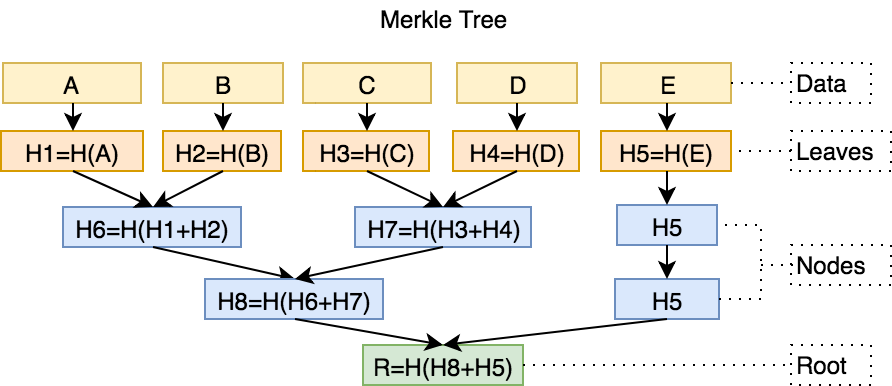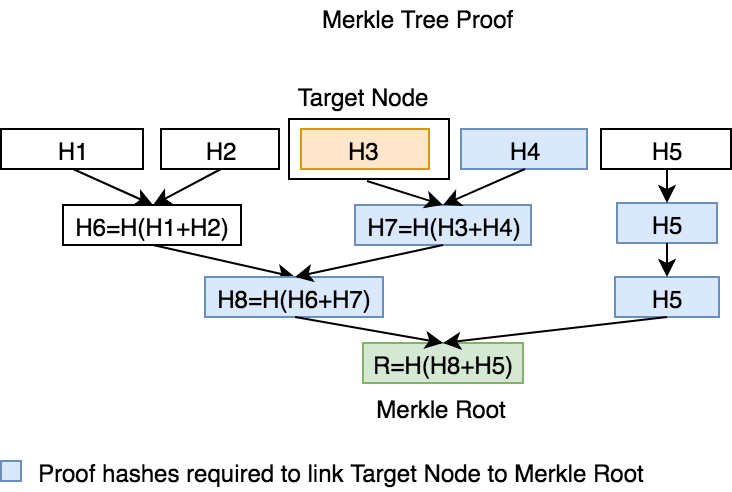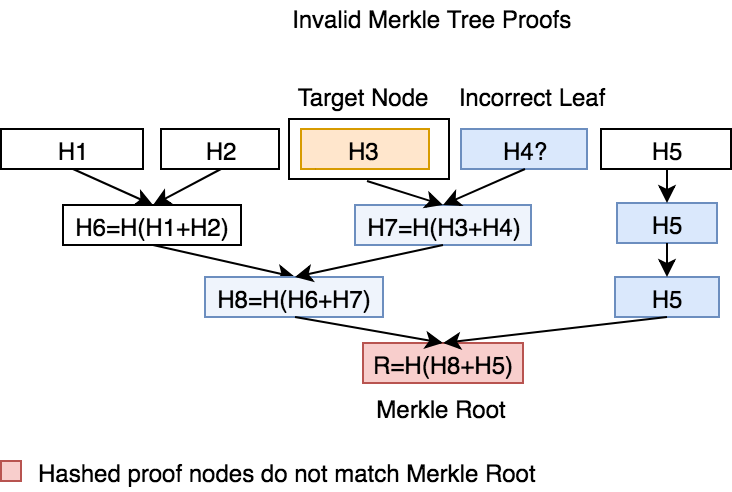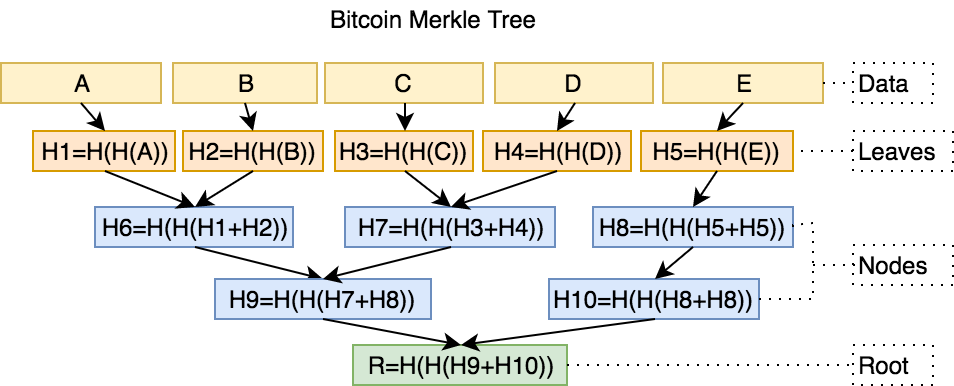# MerkleTree.js

Construct Merkle Trees and verify proofs in JavaScript.

## Install

From NPM:

```npm install merkletreejs
```

### CDN

Available on jsDelivr CDN:

```<script src="https://cdn.jsdelivr.net/npm/[email protected]/merkletree.js"></script>
```

## Getting started

Construct tree, generate proof, and verify proof:

```const { MerkleTree } = require('merkletreejs')
const SHA256 = require('crypto-js/sha256')

const leaves = ['a', 'b', 'c'].map(x => SHA256(x))
const tree = new MerkleTree(leaves, SHA256)
const root = tree.getRoot().toString('hex')
const leaf = SHA256('a')
const proof = tree.getProof(leaf)
console.log(tree.verify(proof, leaf, root)) // true

const badLeaves = ['a', 'x', 'c'].map(x => SHA256(x))
```

Print tree to console:

```MerkleTree.print(tree)
```

Output

```└─ 311d2e46f49b15fff8b746b74ad57f2cc9e0d9939fda94387141a2d3fdf187ae
├─ 176f0f307632fdd5831875eb709e2f68d770b102262998b214ddeb3f04164ae1
│  ├─ 3ac225168df54212a25c1c01fd35bebfea408fdac2e31ddd6f80a4bbf9a5f1cb
│  └─ b5553de315e0edf504d9150af82dafa5c4667fa618ed0a6f19c69b41166c5510
```

## Diagrams

▾ Visualization of Merkle Tree▾ Visualization of Merkle Tree Proof▾ Visualization of Invalid Merkle Tree Proofs▾ Visualization of Bitcoin Merkle Tree# Class: MerkleTree

Class reprensenting a Merkle Tree

`namespace` MerkleTree

• MerkleTree

## Constructors

### constructor

+ new MerkleTree(`leaves`: any[], `hashAlgorithm`: any, `options`: Options): MerkleTree

`desc` Constructs a Merkle Tree. All nodes and leaves are stored as Buffers. Lonely leaf nodes are promoted to the next level up without being hashed again.

`example`

```const MerkleTree = require('merkletreejs')
const crypto = require('crypto')

function sha256(data) {
// returns Buffer
return crypto.createHash('sha256').update(data).digest()
}

const leaves = ['a', 'b', 'c'].map(x => keccak(x))

const tree = new MerkleTree(leaves, sha256)
```

Parameters:

Name Type Default Description
`leaves` any[] - Array of hashed leaves. Each leaf must be a Buffer.
`hashAlgorithm` any SHA256 Algorithm used for hashing leaves and nodes
`options` Options {} Additional options

Returns: MerkleTree

## Methods

### bufferToHex

bufferToHex(`value`: Buffer): string

bufferToHex

`desc` Returns a hex string with 0x prefix for given buffer.

`example`

```const hexStr = tree.bufferToHex(Buffer.from('A'))
```

Parameters:

Name Type
`value` Buffer

Returns: string

### bufferify

bufferify(`value`: any): Buffer

bufferify

`desc` Returns a buffer type for the given value.

`example`

```const buf = tree.bufferify('0x1234')
```

Parameters:

Name Type
`value` any

Returns: Buffer

### getDepth

getDepth(): number

getDepth

`desc` Returns the tree depth (number of layers)

`example`

```const depth = tree.getDepth()
```

Returns: number

### getHexLayers

getHexLayers(): string[]

getHexLayers

`desc` Returns multi-dimensional array of all layers of Merkle Tree, including leaves and root as hex strings.

`example`

```const layers = tree.getHexLayers()
```

Returns: string[]

### getHexLayersFlat

getHexLayersFlat(): string[]

getHexLayersFlat

`desc` Returns single flat array of all layers of Merkle Tree, including leaves and root as hex string.

`example`

```const layers = tree.getHexLayersFlat()
```

Returns: string[]

### getHexLeaves

getHexLeaves(): string[]

getHexLeaves

`desc` Returns array of leaves of Merkle Tree as hex strings.

`example`

```const leaves = tree.getHexLeaves()
```

Returns: string[]

### getHexMultiProof

getHexMultiProof(`tree`: Buffer[], `indices`: number[]): string[]

getHexMultiProof

`desc` Returns the multiproof for given tree indices as hex strings.

`example`

```const indices = [2, 5, 6]
const proof = tree.getHexMultiProof(indices)
```

Parameters:

Name Type Description
`tree` Buffer[] -
`indices` number[] Tree indices.

Returns: string[]

• Multiproofs as hex strings.

### getHexProof

getHexProof(`leaf`: Buffer, `index?`: number): string[]

getHexProof

`desc` Returns the proof for a target leaf as hex strings.

`example`

```const proof = tree.getHexProof(leaves)
```

Parameters:

Name Type Description
`leaf` Buffer Target leaf
`index?` number -

Returns: string[]

• Proof array as hex strings.

### getHexRoot

getHexRoot(): string

getHexRoot

`desc` Returns the Merkle root hash as a hex string.

`example`

```const root = tree.getHexRoot()
```

Returns: string

### getLayers

getLayers(): Buffer[]

getLayers

`desc` Returns multi-dimensional array of all layers of Merkle Tree, including leaves and root.

`example`

```const layers = tree.getLayers()
```

Returns: Buffer[]

### getLayersAsObject

getLayersAsObject(): any

getLayersAsObject

`desc` Returns the layers as nested objects instead of an array.

`example`

```const layersObj = tree.getLayersAsObject()
```

Returns: any

### getLayersFlat

getLayersFlat(): Buffer[]

getLayersFlat

`desc` Returns single flat array of all layers of Merkle Tree, including leaves and root.

`example`

```const layers = tree.getLayersFlat()
```

Returns: Buffer[]

### getLeaves

getLeaves(`values?`: any[]): Buffer[]

getLeaves

`desc` Returns array of leaves of Merkle Tree.

`example`

```const leaves = tree.getLeaves()
```

Parameters:

Name Type
`values?` any[]

Returns: Buffer[]

### getMultiProof

getMultiProof(`tree?`: any[], `indices?`: any[]): Buffer[]

getMultiProof

`desc` Returns the multiproof for given tree indices.

`example`

```const indices = [2, 5, 6]
const proof = tree.getMultiProof(indices)
```

Parameters:

Name Type Description
`tree?` any[] -
`indices?` any[] Tree indices.

Returns: Buffer[]

• Multiproofs

### getProof

getProof(`leaf`: Buffer, `index?`: number): any[]

getProof

`desc` Returns the proof for a target leaf.

`example`

```const proof = tree.getProof(leaves)
```

`example`

```const leaves = ['a', 'b', 'a'].map(x => keccak(x))
const tree = new MerkleTree(leaves, keccak)
const proof = tree.getProof(leaves, 2)
```

Parameters:

Name Type Description
`leaf` Buffer Target leaf
`index?` number -

Returns: any[]

• Array of objects containing a position property of type string with values of 'left' or 'right' and a data property of type Buffer.

### getProofFlags

getProofFlags(`leaves`: Buffer[], `proofs`: Buffer[]): boolean[]

getProofFlags

`desc` Returns list of booleans where proofs should be used instead of hashing. Proof flags are used in the Solidity multiproof verifiers.

`example`

```const indices = [2, 5, 6]
const proof = tree.getMultiProof(indices)
const proofFlags = tree.getProofFlags(leaves, proof)
```

Parameters:

Name Type
`leaves` Buffer[]
`proofs` Buffer[]

Returns: boolean[]

• Boolean flags

### getProofIndices

getProofIndices(`treeIndices`: number[], `depth`: number): number[]

getProofIndices

`desc` Returns the proof indices for given tree indices.

`example`

```const proofIndices = tree.getProofIndices([2,5,6], 4)
console.log(proofIndices) // [ 23, 20, 19, 8, 3 ]
```

Parameters:

Name Type Description
`treeIndices` number[] Tree indices
`depth` number Tree depth; number of layers.

Returns: number[]

• Proof indices

### getRoot

getRoot(): Buffer

getRoot

`desc` Returns the Merkle root hash as a Buffer.

`example`

```const root = tree.getRoot()
```

Returns: Buffer

### print

print(): void

print

`desc` Prints out a visual representation of the merkle tree.

`example`

```tree.print()
```

Returns: void

toJSON(): void

Returns: void

### toString

toString(): string

toString

`desc` Returns a visual representation of the merkle tree as a string.

`example`

```console.log(tree.toString())
```

Returns: string

### verify

verify(`proof`: any[], `targetNode`: Buffer, `root`: Buffer): boolean

verify

`desc` Returns true if the proof path (array of hashes) can connect the target node to the Merkle root.

`example`

```const root = tree.getRoot()
const proof = tree.getProof(leaves)
const verified = tree.verify(proof, leaves, root)
```

Parameters:

Name Type Description
`proof` any[] Array of proof objects that should connect target node to Merkle root.
`targetNode` Buffer Target node Buffer
`root` Buffer Merkle root Buffer

Returns: boolean

### verifyMultiProof

verifyMultiProof(`root`: Buffer, `indices`: number[], `leaves`: Buffer[], `depth`: number, `proof`: Buffer[]): boolean

verifyMultiProof

`desc` Returns true if the multiproofs can connect the leaves to the Merkle root.

`example`

```const root = tree.getRoot()
const treeFlat = tree.getLayersFlat()
const depth = tree.getDepth()
const indices = [2, 5, 6]
const proofLeaves = indices.map(i => leaves[i])
const proof = tree.getMultiProof(treeFlat, indices)
const verified = tree.verifyMultiProof(root, indices, proofLeaves, depth, proof)
```

Parameters:

Name Type Description
`root` Buffer Merkle tree root
`indices` number[] Leave indices
`leaves` Buffer[] Leaf values at indices.
`depth` number Tree depth
`proof` Buffer[] Multiproofs given indices

Returns: boolean

### `Static` bufferToHex

bufferToHex(`value`: Buffer): string

bufferToHex

`desc` Returns a hex string with 0x prefix for given buffer.

`example`

```const hexStr = MerkleTree.bufferToHex(Buffer.from('A'))
```

Parameters:

Name Type
`value` Buffer

Returns: string

### `Static` bufferify

bufferify(`value`: any): Buffer

bufferify

`desc` Returns a buffer type for the given value.

`example`

```const buf = MerkleTree.bufferify('0x1234')
```

Parameters:

Name Type
`value` any

Returns: Buffer

### `Static` getMultiProof

getMultiProof(`tree`: Buffer[], `indices`: number[]): Buffer[]

getMultiProof

`desc` Returns the multiproof for given tree indices.

`example`

```const flatTree = tree.getLayersFlat()
const indices = [2, 5, 6]
const proof = MerkleTree.getMultiProof(flatTree, indices)
```

Parameters:

Name Type Description
`tree` Buffer[] Tree as a flat array.
`indices` number[] Tree indices.

Returns: Buffer[]

• Multiproofs

### `Static` isHexString

isHexString(`v`: string): boolean

isHexString

`desc` Returns true if value is a hex string.

`example`

```console.log(MerkleTree.isHexString('0x1234'))
```

Parameters:

Name Type
`v` string

Returns: boolean

### `Static` marshalLeaves

marshalLeaves(`leaves`: any[]): string

marshalLeaves

`desc` Returns array of leaves of Merkle Tree as a JSON string.

`example`

```const jsonStr = MerkleTree.marshalLeaves(leaves)
```

Parameters:

Name Type
`leaves` any[]

Returns: string

• List of leaves as JSON string

### `Static` marshalProof

marshalProof(`proof`: any[]): string

marshalProof

`desc` Returns proof array as JSON string.

`example`

```const jsonStr = MerkleTree.marshalProof(proof)
```

Parameters:

Name Type Description
`proof` any[] Merkle tree proof array

Returns: string

• Proof array as JSON string.

### `Static` print

print(`tree`: any): void

print

`desc` Prints out a visual representation of the given merkle tree.

`example`

```MerkleTree.print(tree)
```

Parameters:

Name Type Description
`tree` any Merkle tree instance.

Returns: void

### `Static` unmarshalLeaves

unmarshalLeaves(`jsonStr`: string | object): Buffer[]

unmarshalLeaves

`desc` Returns array of leaves of Merkle Tree as a Buffers.

`example`

```const leaves = MerkleTree.unmarshalLeaves(jsonStr)
```

Parameters:

Name Type
`jsonStr` string | object

Returns: Buffer[]

• Unmarshalled list of leaves

### `Static` unmarshalProof

unmarshalProof(`jsonStr`: string | object): any[]

unmarshalProof

`desc` Returns the proof for a target leaf as a list of Buffers.

`example`

```const proof = MerkleTree.unmarshalProof(jsonStr)
```

Parameters:

Name Type
`jsonStr` string | object

Returns: any[]

• Marshalled proof

# Interface: Options

• Options

## Properties

### `Optional` duplicateOdd

duplicateOdd? : boolean

If set to `true`, an odd node will be duplicated and combined to make a pair to generate the layer hash.

### `Optional` hashLeaves

hashLeaves? : boolean

If set to `true`, the leaves will hashed using the set hashing algorithms.

### `Optional` isBitcoinTree

isBitcoinTree? : boolean

If set to `true`, constructs the Merkle Tree using the Bitcoin Merkle Tree implementation. Enable it when you need to replicate Bitcoin constructed Merkle Trees. In Bitcoin Merkle Trees, single nodes are combined with themselves, and each output hash is hashed again.

### `Optional` sort

sort? : boolean

If set to `true`, the leaves and hashing pairs will be sorted.

### `Optional` sortLeaves

sortLeaves? : boolean

If set to `true`, the leaves will be sorted.

### `Optional` sortPairs

sortPairs? : boolean

If set to `true`, the hashing pairs will be sorted.

## Test

```npm test
```

## FAQ

• Q: How do you verify merkle proofs in Solidity?

• A: Check out the example repo merkletreejs-solidity on how to generate merkle proofs with this library and verify them in Solidity.
• Q: How do you verify merkle multiproofs in Solidity?

## Notes

Get A Weekly Email With Trending Projects For These Topics
No Spam. Unsubscribe easily at any time.
javascript (66,554
nodejs (3,505
blockchain (749
ethereum (657
bitcoin (514
algorithm (464
solidity (233
protocol (177
smart-contracts (142
hashing (51
merkle-tree (24